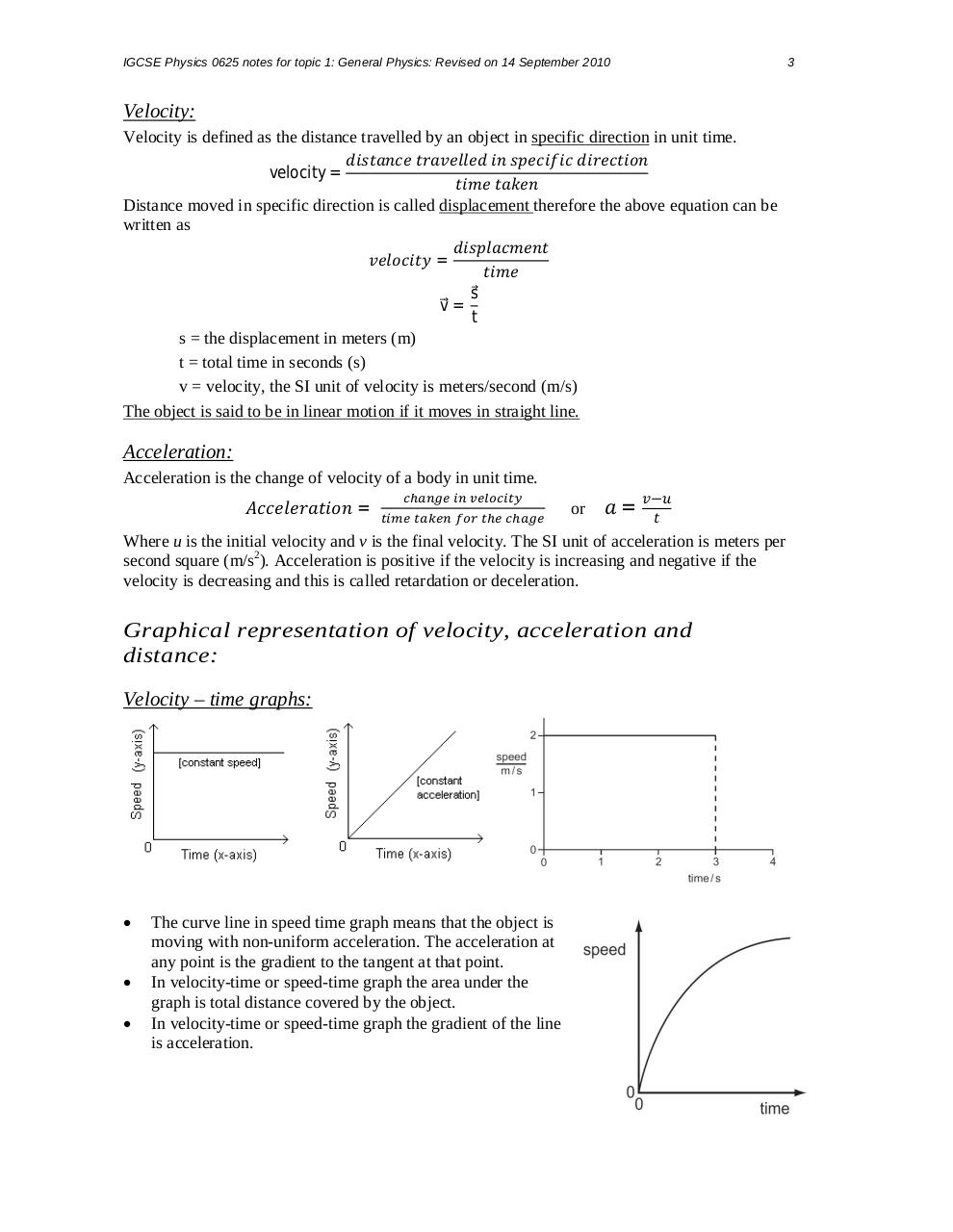# Summary of Chapter 2,3,4.pdfPage 1 2 34517

#### Text preview

IGCSE Physics 0625 notes for topic 1: General Physics: Revised on 14 September 2010

3

Velocity:
Velocity is defined as the distance travelled by an object in specific direction in unit time.
velocity =
Distance moved in specific direction is called displacement therefore the above equation can be
written as
=
v⃗ =

s⃗
t

s = the displacement in meters (m)
t = total time in seconds (s)
v = velocity, the SI unit of velocity is meters/second (m/s)
The object is said to be in linear motion if it moves in straight line.

Acceleration:
Acceleration is the change of velocity of a body in unit time.

=

or

=

Where u is the initial velocity and v is the final velocity. The SI unit of acceleration is meters per
second square (m/s2). Acceleration is positive if the velocity is increasing and negative if the
velocity is decreasing and this is called retardation or deceleration.

Graphical representation of velocity, acceleration and
distance:
Velocity – time graphs:

The curve line in speed time graph means that the object is
moving with non-uniform acceleration. The acceleration at
any point is the gradient to the tangent at that point.
In velocity-time or speed-time graph the area under the
graph is total distance covered by the object.
In velocity-time or speed-time graph the gradient of the line
is acceleration.